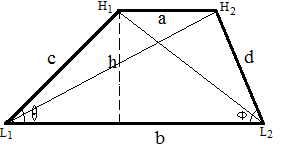## Trapezoid Calculator

 Parallel Side a: Parallel Side b: Side c: Side d: Altitude (h): Area: Perimeter: Diagonal L1H2: Diagonal H1L2: Angle (θ): radian  ̊ degree  π  π/3 Angle (Φ) radian  ̊ degree  π  π/3

In geometry, A trapezoid has two parallel sides are called the bases of the trapezoid. The other two sides are calld the lateral sides.

### Trapezoid Formulas1. Area = h(a+b)/2

2. Perimeter = a + b + c + d

3. Diagonal L1H2 = sqrt(h2+(b-sqrt(c2-h2))2)

4. Diagonal H1L2 = sqrt(h2+(b-sqrt(d2-h2))2)

For example, When Parallel Side a =3, b = 6, Side c = d = 4, altitude(h) = 3, Then the area= 13.5, perimeter = 17, Diagonal L1H2 = 4.5, Diagonal H1L2 = 4.5, Angle (θ) = Angle (Φ) = 0.8481

Thinkcalculator.com provides you helpful and handy calculator resources.